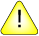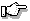This version of this document is no longer maintained. For the latest documentation, see http://www.qnx.com/developers/docs.

sqrt(), sqrtf()

Calculate the nonnegative square root of a number

Synopsis:

#include <math.h>

double sqrt( double x );

float sqrtf( float x );

Arguments:

x
The number that you want to calculate the square root of.

Library:

libm

Use the -l m option to qcc to link against this library.

Description:

These functions compute the nonnegative square root of x. A domain error occurs if the argument is negative.

Returns:

The nonnegative square root of the given number.If an error occurs, these functions return 0, but this is also a valid mathematical result. If you want to check for errors, set errno to 0, call the function, and then check errno again. These functions don't change errno if no errors occurred.

Examples:

#include <stdio.h>
#include <math.h>
#include <stdlib.h>

int main( void )
{
printf( "%f\n", sqrt(.5) );
return EXIT_SUCCESS;
}

produces the output:

0.707107

Classification:

Safety:
Cancellation point No
Interrupt handler No
Signal handler No